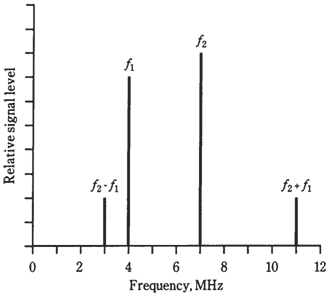## Heterodyning Assignment Help

Assignment Help: >> Electrical Engineering - Heterodyning

Heterodyning:

When two waves which are having different frequencies are combined in a nonlinear circuit, new frequencies are obtained. These new waves are at sum and difference frequencies of original waves. You have probably noticed this mixing, called as heterodyning, if you have ever heard two loud, sine wave tones at same time.

Assume that there are two signals having frequencies fl and f2. For the mathematical convenience, assign f2 to wave with higher frequency. If these signals are combined in the nonlinear circuit, new waves will appear. One of them will have a frequency f2 - fl, and the other will be at f2 + f1. These are called as beat frequencies. The signals are called as mixing products.Figure   Spectral (frequency-domain)  illustration of mixing.

Figure given above is a frequency domain graph. Amplitude (on vertical scale) is shown as the function of frequency (on horizontal scale). This kind of display is seen by engineers when they look at the screen of the spectrum analyzer. Most of the graphs you have seen so far have been time domain graphs, in which things are shown as function of time. The screen of the oscilloscope normally shows things in time domain.

How do you get nonlinearity necessary to obtain the mixer circuit? There are different schemes, but one common way is to use the diodes.